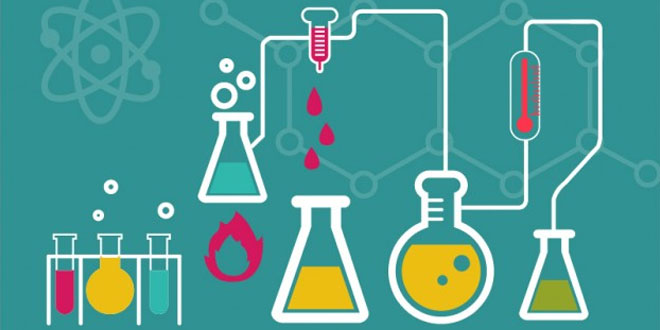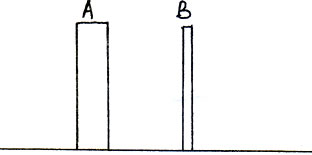Friday , September 30 2022# 8th Science Periodic Test II (2017-18)

## 8th Science Periodic Test II (2017-18)

School Name: Delhi Public School, Sector 24, Rohini, Delhi 110085 India
Time: 1 hour
M.M. 30 marks
Date: 26/07/2017
Class: VIII

#### Question: 1. (1×5 = 5)

1. A ball of dough is rolled into a flat chapatti. Name the force exerted to change the shape of the dough.
2. Name a non metal which is a good conductor of electricity.
3. A girl is pushing a box towards east direction. In which direction should her friend push the box so that it moves faster in the same direction?
4. A non-metal X in gaseous form is greenish yellow in colour and is used in water purification. Identify the non metal.
5. Define the term Malleability.

#### Question: Choose the correct option: (1×3 = 3) (I). Two forces A and B act on an object in opposite directions. A is bigger than B. The net force on the object is

1. A+B acting in the direction of A
2. A-B acting in the direction of B
3. A+B acting in the direction of B
4. A-B acting in the direction of A

#### (II). Which of the following does not change when a force acts on an object?

1. Direction of motion
2. State of rest
3. Shape
4. mass

1. Ca(SO3)2
2. CaSO3
3. CaSO4
4. Ca(SO4)2

#### Question: 3. Two rods of the same weight and equal length have different thickness. They are held vertically on the surface of sand as shown in figure. Which one of them will sink more Why? (2 marks)#### Question: 5. (2 marks)

1. What is meant by atmospheric pressure?
2. We do not get crushed the huge atmospheric pressure. Why?

#### Question: 6. Give reasons: (2 marks)

1. The wheels of military tanks are covered with a broad rubber strip.
2. Electrical wires are made up of metals like aluminium and copper.

#### Question: 7. (3 marks)

1. Define pressure.
2. A man standing on a wooden plank 100 cm long and 40 cm wide exerts a force of 60 N. Calculate the pressure on the ground.

#### Question: 8. (3 marks)

1. Define corrosion
2. State the conditions necessary for corrosion of copper to take place.
3. Write the chemical equation for correction of copper.

#### Question: 9. Complete and balance the following equations: (3 marks)

1. Al + H2SO4  →
2. Ag + H2S →

#### Question: 10. (a). A small piece of sodium metal was introduced into a beaker containing cold water. A vigorous reaction with evolution of fumes was observed in the beaker. After the reaction, the solution in the beaker was tested with a red litmus paper. (5 marks)

1. Which gas was given out during the reaction?
2. What change would be observed in the red litmus paper?
3. Write the balanced chemical equation for the reaction of sodium with water.

#### (b). Rohan prepared a blue colored solution of copper sulphate in beaker A and placed an iron nail in it. Swati prepared a pale green solution of ferrous sulphate in breaker B and placed a copper wire in it.

1. What changes will they observe in the two beakers after an hour? Give reasons for you answer.
2. Write chemical equation for the reactions taking place.
3. What are such reactions called?

## 11th Class English Term 2 Question Paper 2021-22

School Name: Himalaya Public School, Sector 13, Rohini, Delhi 110085 India Class: 11th Standard (CBSE) Subject: English Time …